### Home > PC > Chapter 4 > Lesson 4.3.2 > Problem4-142

4-142.
1. Find reasonable equations for each graph below. Homework Help ✎

1.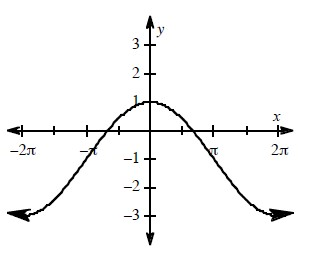2.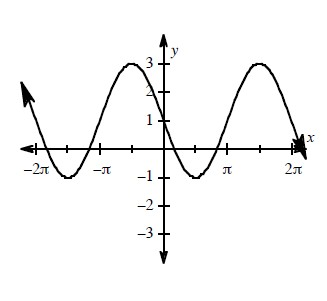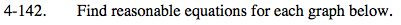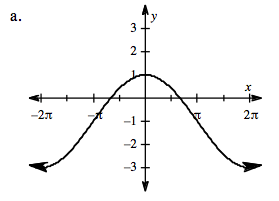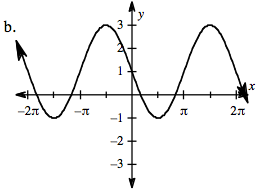This is the cosine function:
1. Where is the midline?
2. What is the amplitude?
3. Identify the period and calculate the frequency.

Substitute the information in the general form for the cosine.

This is the sine function:
1. Where is the midline?
2. What is the amplitude?
3. Identify the period and calculate the frequency.

Substitute the information in the general form for the sine.

Use the eTool below to visualize this problem.
Click on the link to the right to view the full version of the eTool: PCT 4-142 eTool